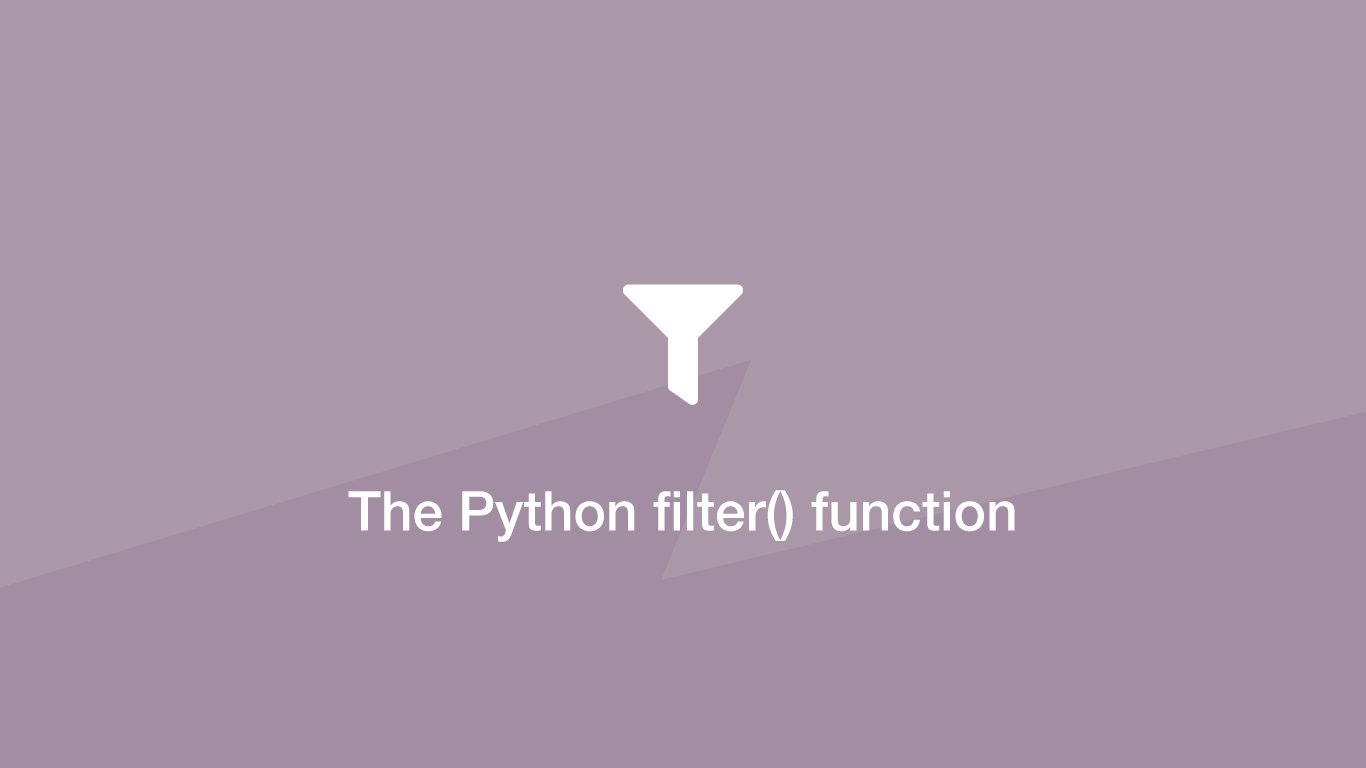# How to use the Python filter() FunctionThe Python `filter()` function is used to iterate through data and return a new iterable containing elements that matched a given condition. Typically the `filter()` function will use a function to determine whether a value exists in the iterable.

In this tutorial, we will learn how to use the Python `filter()` method with examples.

## filter() Syntax

`filter()` has two required parameters, firstly the function used to check for a value and secondly the iterable data to use.

``````result = filter(function, iterable)
``````

• `function` - a function that will look for a value in the iterable and return `True` if a match is found (can be set to None).
• `iterable` - the data to loop through this could be a set, dictionary, list or string.

## Filter a list

Let's filter a `list`. We will do this by creating a new function to check if a value exists in a list of data and return `True` if a match was found. In this function, there will be a list containing values that should be kept.

``````fruit = ['orange', 'apple', 'strawberry', 'apricot']

def check_exists(value):
keep = ['apple', 'apricot']

if value in keep:
return True
else:
return False

result = filter(check_exists, fruit)

for r in result:
print(r)
``````
``````apple
apricot
``````

In the `filter()` function we calling `check_exists()` on each iteration of the `fruit` list and passing it the value to check. Inside `check_exists()` the `keep` variable stores a list of values that should be kept; if the value is in `keep` `True` is returned, which tells `filter()` to add that value to the new filtered object.

## Remove False Values from an Iterable

To remove all `False` values from a `list` we can use the `filter()` function, except instead of supplying a function as the first parameter we will simply supply `None`.

``````stuff = [False, 'false', '0', 0, True, 'true', '1', 1]
result = filter(None, stuff)

for r in result:
print(r)
``````
``````false
0
True
true
1
1
``````

Notice only the actual `False` value and boolean false (`0`) is removed, not the falsy values in strings.

## Conclusion

You now know how to use the Python filter() function to filter iterables in two different ways.